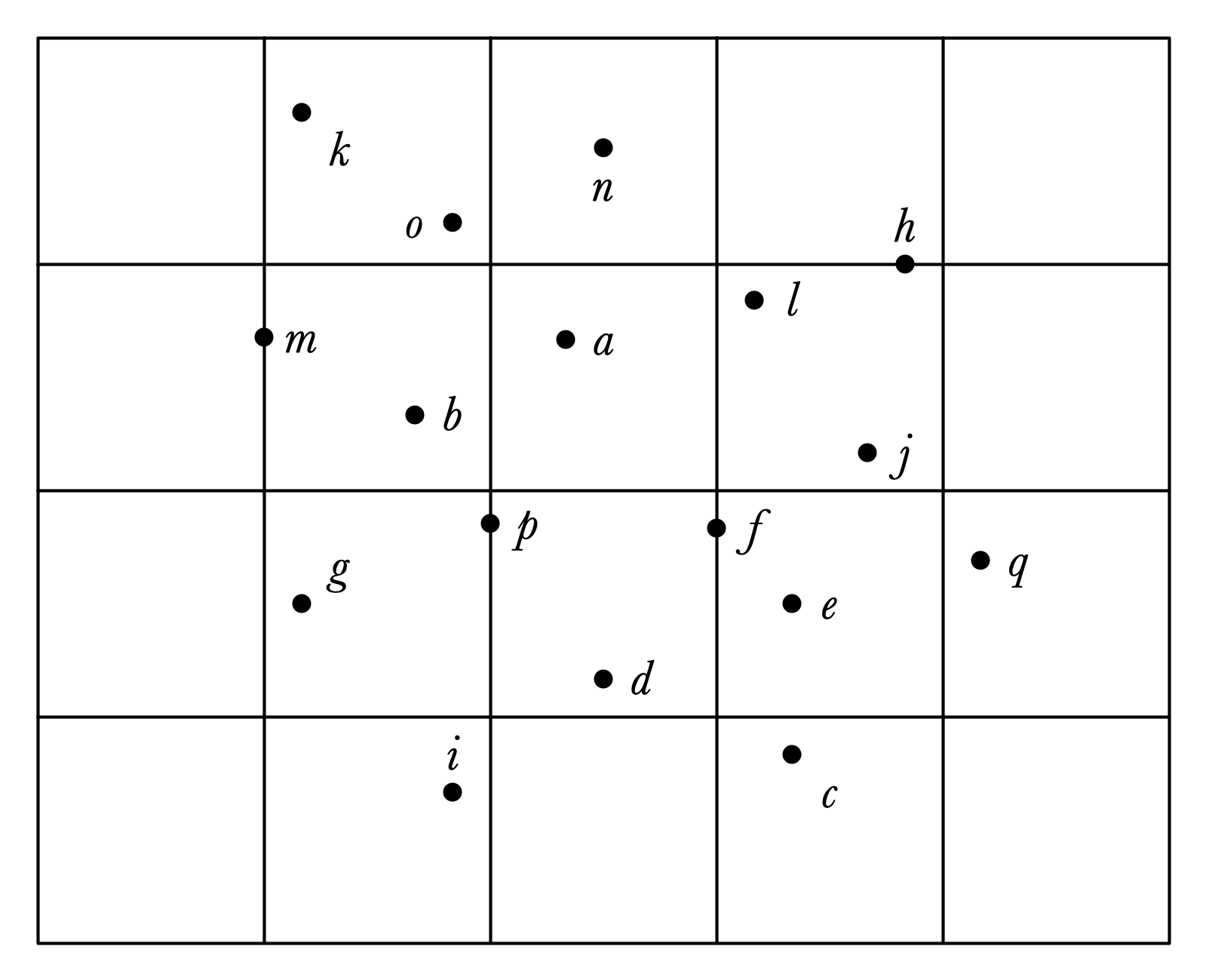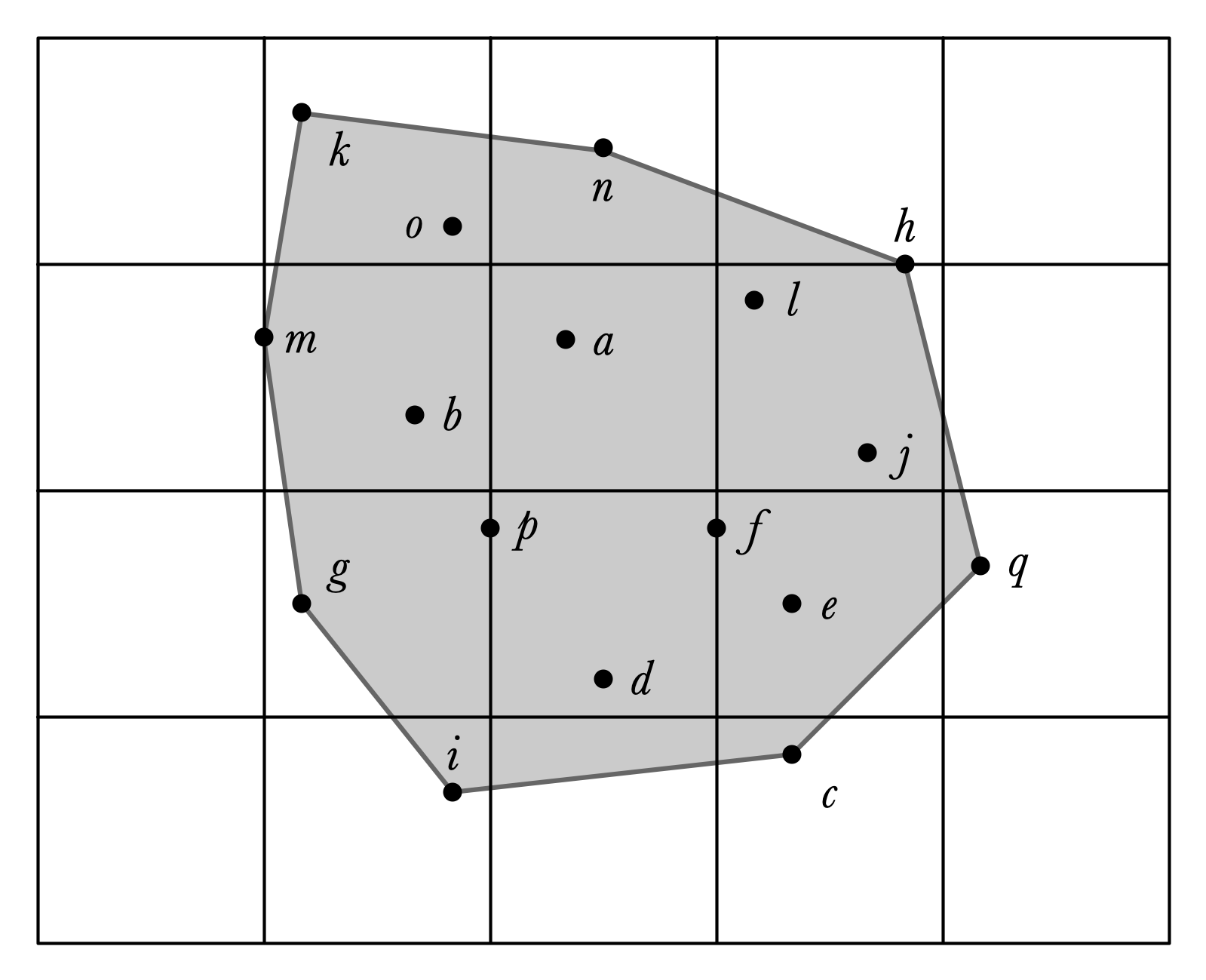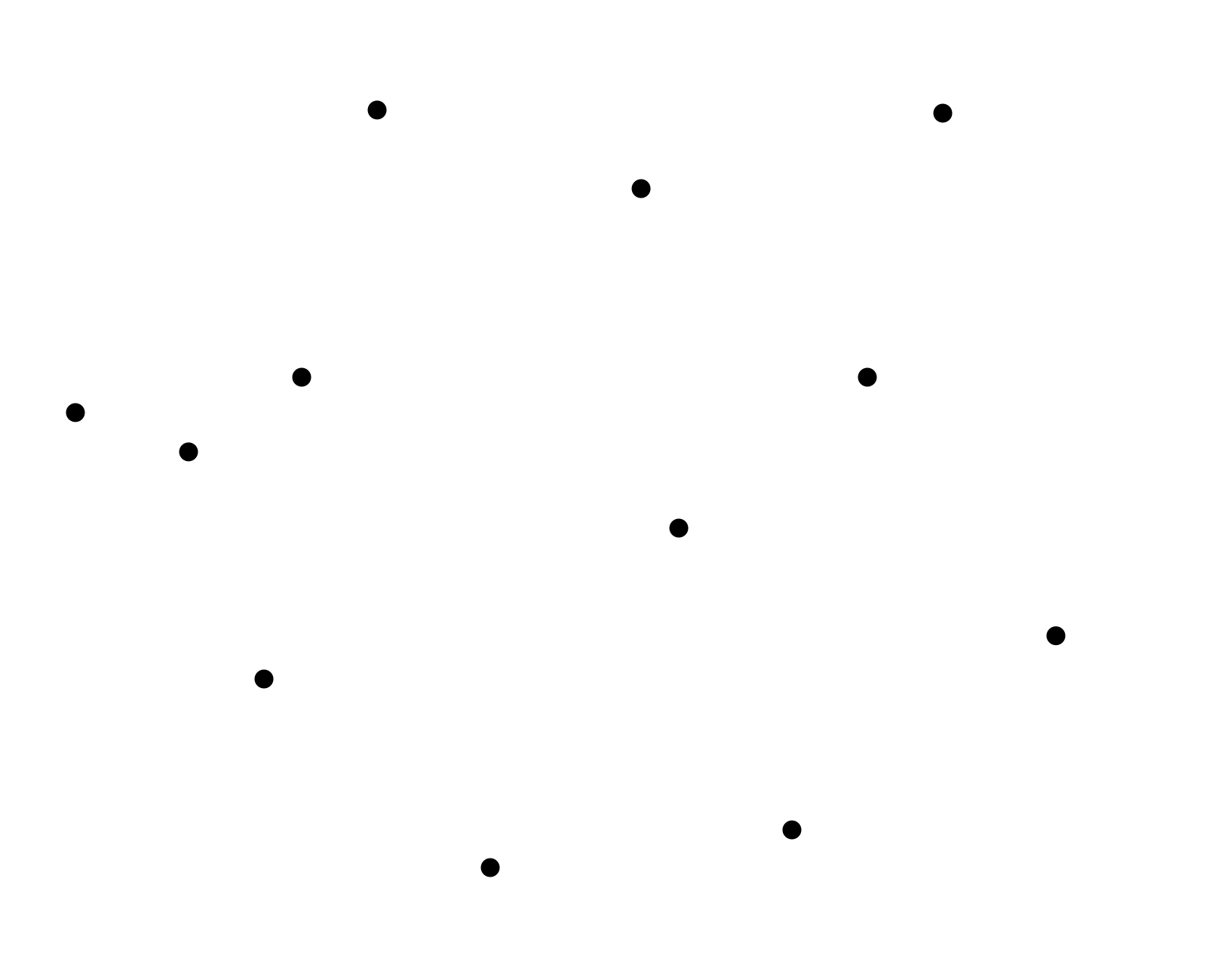# Lecture 10: Convex Hulls; Animation

## Annoucements

Assignment 06: Submit Pair Preferecnes Today!

## Outline

1. Convex Hulls, Finished
2. 3 Ways to Animate!
• CSS transition property
• setInterval
• window.requestAnimationFrame

## Last Time: Convex Hulls

Input:

• set of points in plane
• $(x, y)$-coordinates of each point

Output:

• a sequence of points $(x_1, y_1), (x_2, y_2), \ldots, (x_k, y_k)$ that define the “boundary” of the set of points
• path around $(x_1, y_1), (x_2, y_2), \ldots, (x_k, y_k)$ surrounds all points in the set in clockwise order
• the bounded region is convex

## Input## Convex Hull## Grahm’s Scan Algorithm

1. Pre-process $X$ by sorting by $x$-coordinate:
2. Consider points one by one to determine if they are on the upper boundary of $CH(X)$
3. repeat process from right to left to get lower convex hull

## Graham Scan Idea, Illustrated## Graham’s Scan Pseudocode

• X sorted by $x$-coordinate
• stk a stack, initially storing first two points in X

For each remaining C in X:

• if stk.size() == 1, stk.push(C)
• otherwise
• A and B are top two elements in stk
• while ABC is not a right turn and stk.size() > 1
• stk.pop(), update A, B
• stk.push(C)

## Graham Scan with a Stack## Claim

When Graham’s Scan completes, stk stores the points along the upper boundary of the convex hull of $X$.

Why?

Must show:

1. Sequence of points in stk make only right turns.
2. All points in $X$ are below path formed by points in stk

## Popping Moves Boundary Up## Graham’s Scan Efficiency?

If there are $n$ points, what is the running time of Graham’s scan?

• assume: stack operations are $O(1)$, “left turn” is $O(1)$

## Pseudocode Again

• X sorted by $x$-coordinate
• stk a stack, initially storing first two points in X

For each remaining C in X:

• if stk.size() == 1, stk.push(C)
• otherwise
• A and B are top two elements in stk
• while ABC is not a right turn and stk.size() > 1
• stk.pop(), update A, B
• stk.push(C)

## Finishing the Computation

How to find the lower boundary of $CH(X)$?

## Lower Boundary Illustrated## Assignment 06

Make an interactive visualization for Graham’s scan algorithm

• user can add points in the plane
• program steps through execution and illustrates each step
• returns convex hull of points
• separate non-interactive method for testing

Complete description coming soon!

# Animation

## Updated DFS Visualization

1. Now highlights current node.
2. Has an animate button

Demo!

• lec10-dfs-animated.zip

## Highlighted Vertex

GraphVisualizer changes

1. Added new SVG layer: overlayGroup
• <g></g> element for “group”
• sits “above” other layers (edges, vertices)
2. Methods:
• addOverlayVertex(vtx)
• moveOverlayVertex(vtx1, vtx2)
• removeOverlayVertex(vtx)

Dfs changes

• create and move overlay vertex for cur vertex

## A Simple Goal

Goal 1. Show motion of highlighted vertex

• highlight doesn’t “jump” from one frame to the next

## Simple Animation with CSS

For this animation:

• only changes are to two attributes of a circle object
• cx and cy change
• one-shot animation in response to change

Such things can be animated with CSS!

• have class overlay-vertex

## CSS Transitions

/* list the properties to apply transition to */
transition-property: cx, cy;

/* how long should transition last?*/
transition-duration: 500ms;

/* how long
/* some options: ease (default), ease-in, ease-out, ease-in-out, linear*/
transition-timing-function: ease;

/* should we delay the start of the transition? */
transition-delay: 500ms;


## A Less Simple Goal

Goal 2. Animate an entire execution of DFS without manually stepping through.

• How fast should steps be?

## JavaScript Timed Iteration

setInterval(method, time, arg1, ...) method:

• call method with arguments arg1, ... every time ms, until eternity
• returns the ID of an Interval
• to stop, use clearInterval(id) method

The method is called a callback method

## DFS Animation

Repeatedly call step() function until algorithm terminates

I used three methods:

• animate to start the animation
• animateStep to decide what to do for a single animation step
• call step() if the algorithm isn’t done
• stop the animation if it is done
• stopAnimation to stop the animation

## Starting the Animation

    this.animate = function () {
if (this.curAnimation == null) {
this.start();
this.curAnimation = setInterval(() => {
this.animateStep();
}, 1000);
}
}


## Animating a Step

    this.animateStep = function () {
if (this.active.length > 0) {
console.log("taking a step from vertex "
+ this.cur.id);
this.step();
} else {
this.stopAnimation();
}
}


## Stopping the Animation

    this.stopAnimation = function () {
clearInterval(this.curAnimation);
this.curAnimation = null;
console.log("animation completed");
}


## Animation: A Third Way

• lec10-bouncing-ball.zip

Goal. Animate a bunch of dots that bounce around the screen!

• simple movement
• indefinite animation
• no fixed start/end position

## Bouncing Ball Implementation

window.requestAnimationFrame(callback):

• perform operations one time, then redraw screen
• precise timing is set by system
• to animate motion, must call requestAnimationFrame for each frame
• this is typically done by having callback recursively call the function making the request

## Dot Animation Example

Each dot executes this code:

    this.animate = function () {
this.updateLocation(this.cx + this.vx, this.cy + this.vy);
if(this.cx <= 0 || this.cx >= WIDTH) {
this.vx = -this.vx;
}
if(this.cy <= 0 || this.cy >= HEIGHT) {
this.vy = -this.vy;
}
window.requestAnimationFrame(() => this.animate());
}